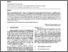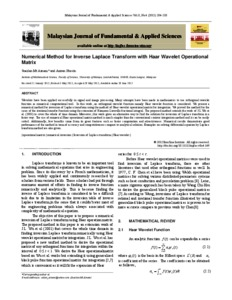# Numerical method for inverse laplace transform with Haar wavelet operational matrix

Mt Aznam, Suazlan and Hussin, Amran (2012) Numerical method for inverse laplace transform with Haar wavelet operational matrix. Malaysian Journal of Fundamental and Applied Sciences, 8 (4 (October-December)). pp. 204-210. ISSN 2289-5981 E-ISSN 2289-599XPreview
PDF - Published Version

## Abstract

Wavelets have been applied successfully in signal and image processing. Many attempts have been made in mathematics to use orthogonal wavelet function as numerical computational tool. In this work, an orthogonal wavelet function namely Haar wavelet function is considered. We present a numerical method for inversion of Laplace transform using the method of Haar wavelet operational matrix for integration. We proved the method for the cases of the irrational transfer function using the extension of Riemenn-Liouville fractional integral. The proposed method extends the work of J.L.Wu et al. (2001) to cover the whole of time domain. Moreover, this work gives an alternative way to find the solution for inversion of Laplace transform in a faster way. The use of numerical Haar operational matrix method is much simpler than the conventional contour integration method and it can be easily coded. Additionally, few benefits come from its great features such as faster computation and attractiveness. Numerical results demonstrate good performance of the method in term of accuracy and competitiveness compare to analytical solution. Examples on solving differential equation by Laplace transform method are also given.

Item Type: Article (Journal) 8062/65471 operational matrix, numerical inversion, Inversion of Laplace transform, Haar wavelet Q Science > QA Mathematics > QA297 Numerical Analysis Kulliyyah of EngineeringKulliyyah of Engineering > Department of Science Dr. Suazlan Mt Aznam 14 Sep 2018 15:17 14 Sep 2018 15:17 http://irep.iium.edu.my/id/eprint/65471View Item